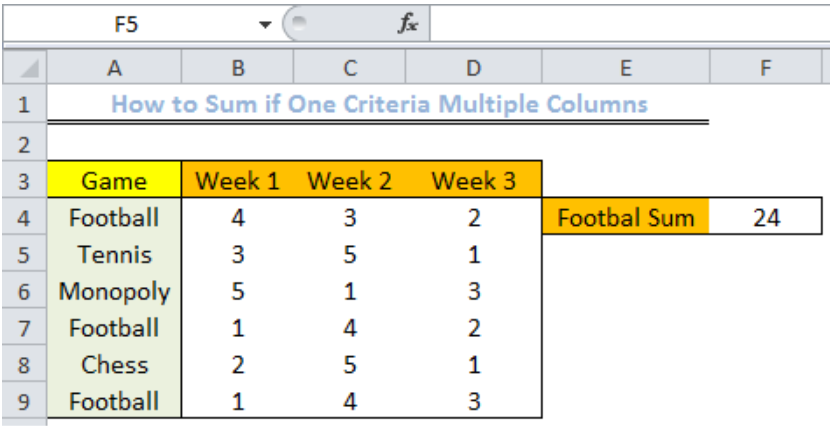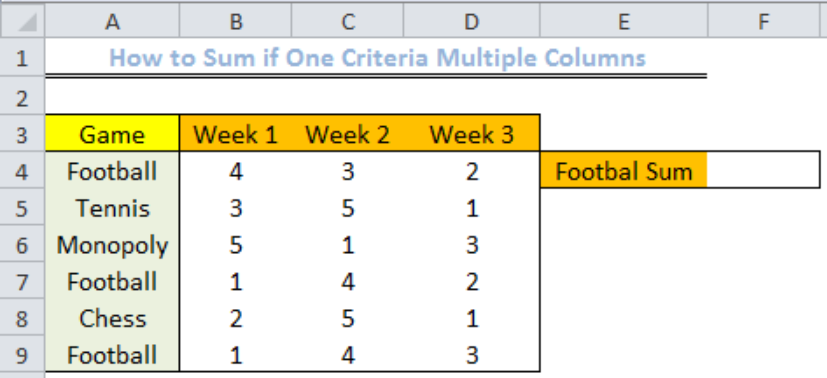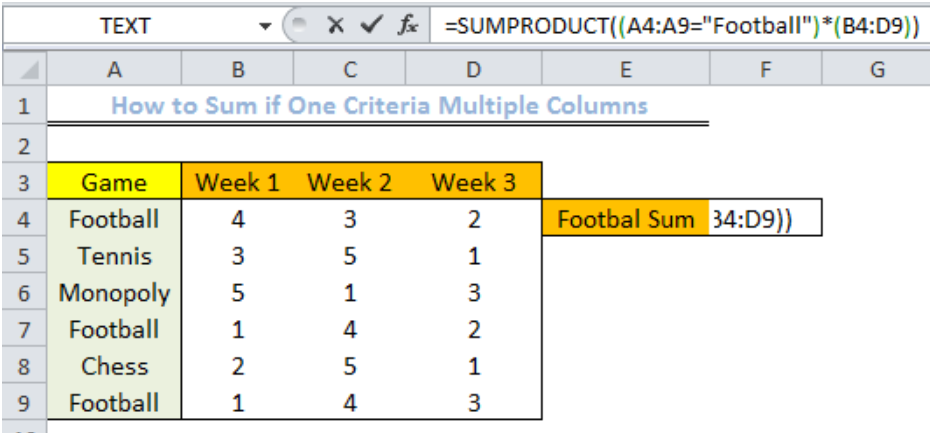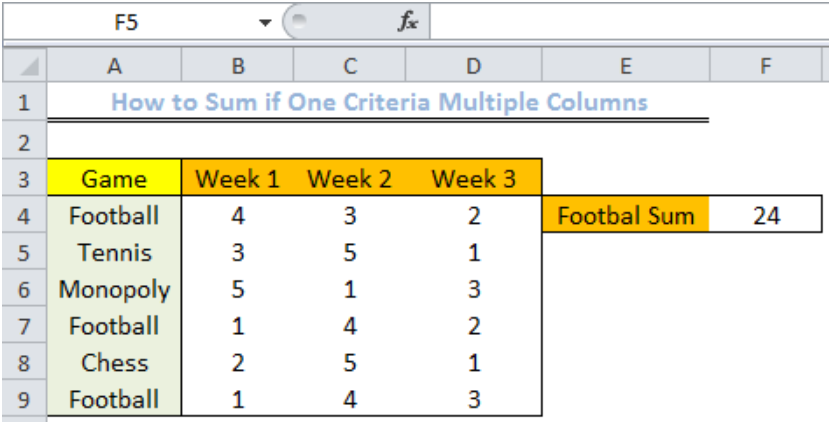Get instant live expert help with Excel or Google Sheets“My Excelchat expert helped me in less than 20 minutes, saving me what would have been 5 hours of work!”

#### Post your problem and you’ll get Expert help in seconds.

Your message must be at least 40 characters
Our professional Expert are available now. Your privacy is guaranteed.

# How to Sum if One Criteria Multiple Columns

We can sum multiple columns conditionally by specifying a criterion. We can do this with a formula that is based on the SUMPRODUCT function. The steps below will walk through the process.Figure 1: Result of the Sum of Multiple Columns with Football as the Criterion

## Basic Formula

`=SUMPRODUCT((criterion_range=”football”)*(sum_range))`

## Formula

`=SUMPRODUCT((A4:A9=”Football”)*(B4:D9))`

## Setting up the Data

• We will set up the data by inserting the values into Column A to Column D
• We will input football sum in Cell E4 as football will be the criterion in this example
• The result will be displayed in Cell F4 which is currently emptyFigure 2: How to Sum if One Criteria Multiple Columns

## Inserting the Formula

• We will click on Cell F4
• We will insert the formula below into the cell

`SUMPRODUCT((A4:A9=”Football”)*(B4:D9))`Figure 3: How to Sum if One Criteria Multiple Columns

• We will click on enterFigure 4: Result of the Sum of Multiple Columns with Football as the Criterion

## Explanation

Formula: `=SUMPRODUCT((A4:A9=”Football”)*(B4:D9))`

In the formula, we use the SUMPRODUCT function to check if Cell A4 to Cell A9 contains “football.” We will have an array result of TRUE or FALSE like this:

`{TRUE;FALSE;FALSE;TRUE;FALSE;FALSE}`

This is multiplied by the values in the range B4:D9, where TRUE represents 1 and FALSE represents 0.

The result inside the SUMPRODUCT function looks like this:

`SUMPRODUCT({4,3,2;0,0,0;0,0,0;1,4,2;0,0,0;1,4,3})`

The product is summed and the result is 24 as shown in figure 4.

## Instant Connection to an Expert through our Excelchat Service

Most of the time, the problem you will need to solve will be more complex than a simple application of a formula or function. If you want to save hours of research and frustration, try our live Excelchat service! Our Excel Experts are available 24/7 to answer any Excel question you may have. We guarantee a connection within 30 seconds and a customized solution within 20 minutes.

### Did this post not answer your question? Get a solution from connecting with the expert.Another blog reader asked this question today on Excelchat:
Solution examplesUse the Vlookup Function to complete the "employee" column of table 2. Use "job Id" from table 2 as your lookup_value(s) and table 1 as your reference.
Solved by C. H. in 16 minsIf a cell in another sheet is populated I need a vlookup done. If the cell is not populated I need the cell to return blank.
Solved by T. D. in 60 minsI am trying to make a chart that turns a week range red if nothing is entered in the range. If something is entered then I would like it to turn green. Please Help
Solved by E. U. in 43 minsI need a check box to show/hide an answer of an if function
Solved by Z. U. in 23 minsI need a formula to compare the data in two columns and then export the mismatched data in the 3rd column
Solved by S. Q. in 20 mins## Subscribe to Excelchat.coAnother blog reader asked this question today on Excelchat: